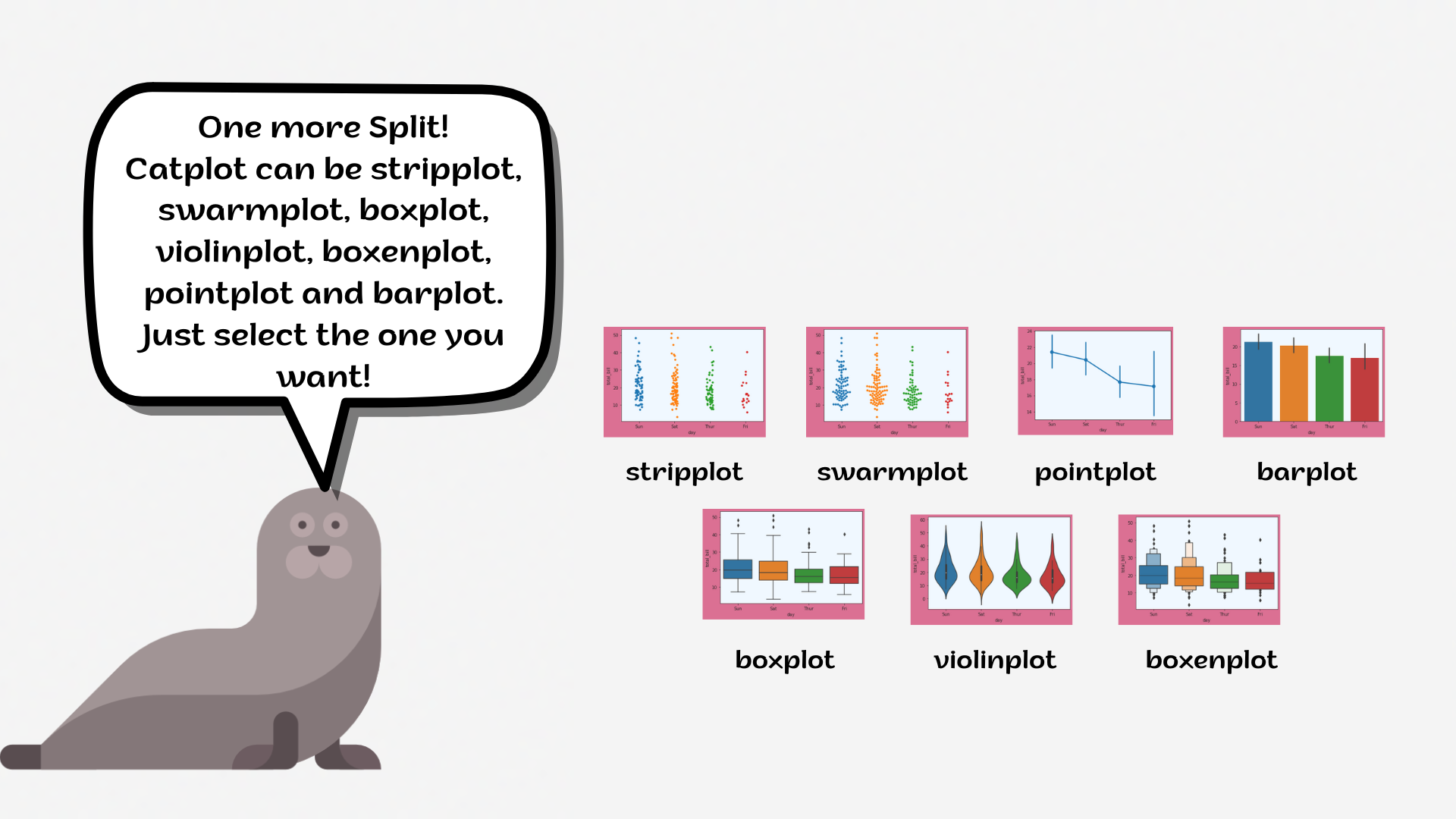Course Content

# Deep Dive into the seaborn Visualization

1. Light Start

2. Distributions of Data

3. Categorical Plot Types

4. Matrix Plots

5. Multi-Plot Grids

6. Regression Models

Deep Dive into the seaborn Visualization

##CatplotNote

`catplot` = `FacetGrid` + `stripplot`/`swarmplot`/`boxplot`/`violinplot`/`barplot`/`pointplot`

The `catplot` function provides a new framework giving access to several types of plots that show a relationship between a numerical variable and one or more categorical variables, like `boxplot`, `stripplot` and so on.Note

Scroll down to see the full plot when you cope with the task!

1. Set the `'white'` style with the `'aliceblue'` `axes.facecolor`.
2. Create the `catplot` using the `seaborn` library:
• Set the `x` parameter equals the `'species'`;
• Set the `y` parameter equals the `'body_mass_g'`;
• Set the `hue` parameter equals the `'sex'`;
• Set the `row` parameter equals the `'island'`;
• Set the `'viridis'` `palette`;
• Set the `alpha` parameter equals `0.6`;
• Disable the `legend_out`.

Everything was clear?

Section 3. Chapter 8